# YOLO algorithm

Last updated on：a year ago

YOLO (you only look once) is a fast detection algorithm, which is widely used in autonomous driving car.

# YOLO

YOLO is an Image classification and localization algorithm. What the YOLO algorithm does is it takes the midpoint of each of the objects and then assigns the object to the grid cell containing the midpoint.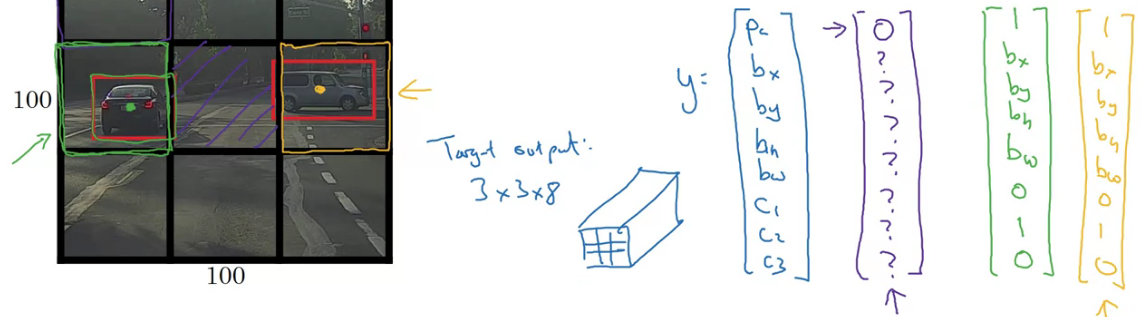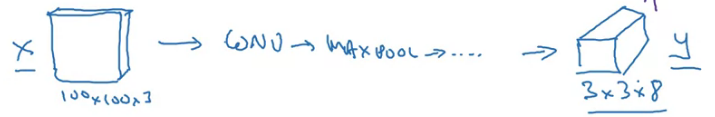For each grid cell:

$$L (\hat{y}, y) = (\hat{y}_1 - y_1)^2 + (\hat{y}_2 - y_2)^2 + … + (\hat{y}_8 - y_8)^2, if y_1 = 1 (\hat{y}_1 - y_1)^2 if y_1 = 0$$

The object is only assigned to one of the grid cells. In practice maybe use $19\times 19 \times 8$ grids.

## Architectures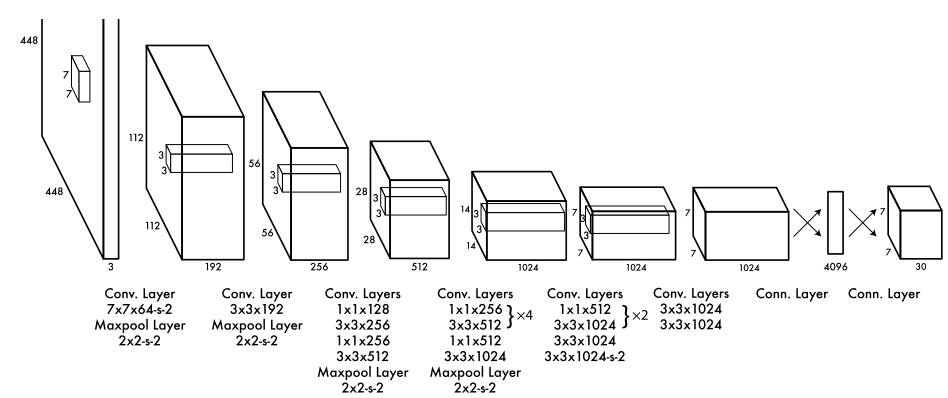• output precise bounding boxes
• output much more precise coordinates that are not just dictated by the stripe size of your sliding windows classifier
• this is a convolutional implementation and you only need to implement this algorithm once
• runs very fast, it works even for real time object detection

## Specify the bounding boxes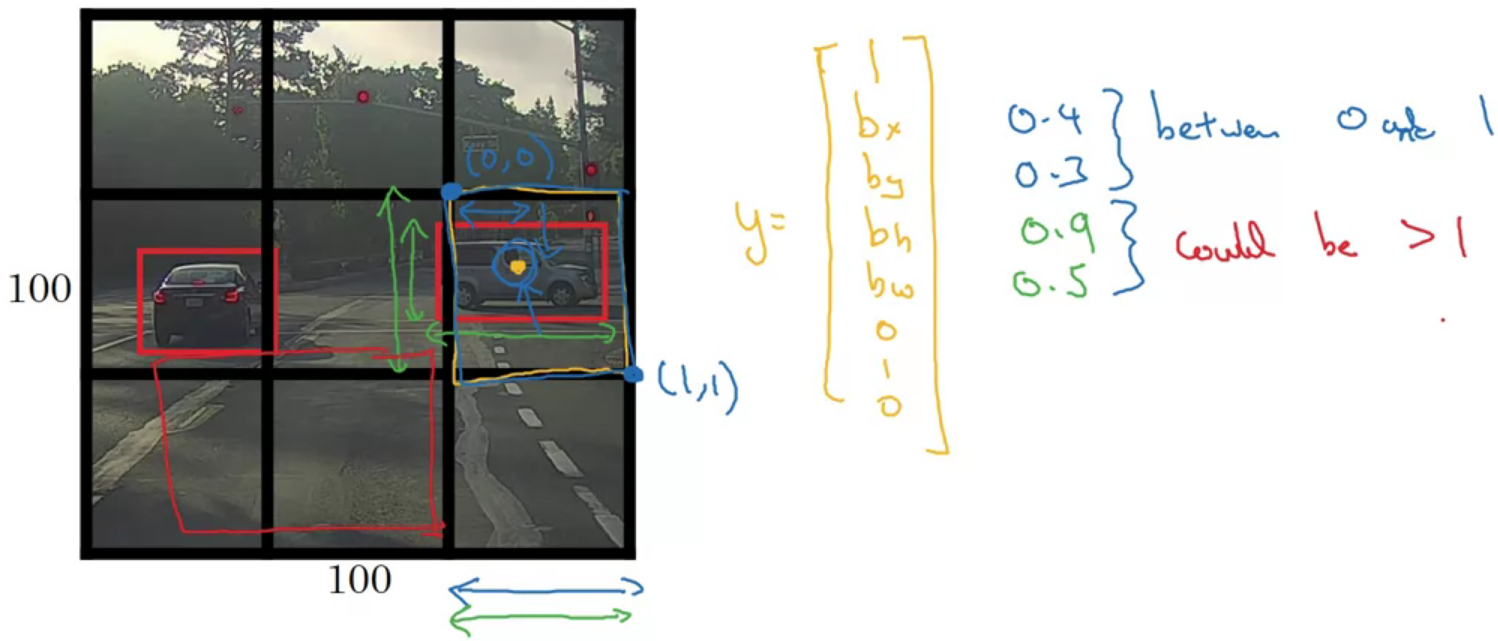## Intersection over union (IoU)

Evaluating object localization. IoU is a measure of the overlap between two bounding boxes. IoU means the ratio of bounding box to ground truth.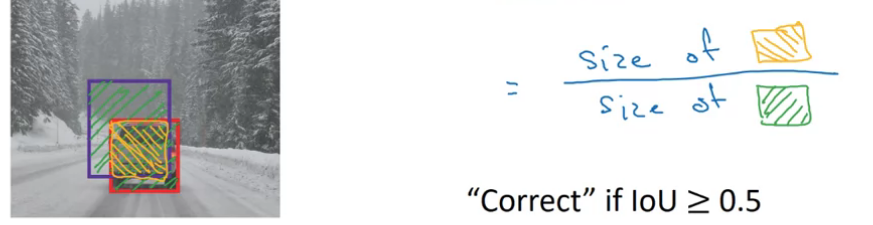## Non-max suppression

$p_c \le 0.6$
While there are any remaining boxes:

• Pick the box with the largest $p_c$ output that as a prediction (just for car detection)

• Discard any remaining box with IoU $\ge 0.5$ with the box output in the previous step

Outputting the non-max supressed outputs

• For each grid call, get 2 predicted bounding boxes
• get rid of low probability predictions
• for each class (pedestrian, car, motorcycle) use non-max suppression to generate final predictions
It is used to remove those boxes which close to the prediction boxes.
Codes:
def nms(self, dets, scores):
""""Pure Python NMS baseline."""
x1 = dets[:, 0]  #xmin
y1 = dets[:, 1]  #ymin
x2 = dets[:, 2]  #xmax
y2 = dets[:, 3]  #ymax

areas = (x2 - x1) * (y2 - y1)
order = scores.argsort()[::-1]

keep = []
while order.size > 0:
i = order
keep.append(i)
xx1 = np.maximum(x1[i], x1[order[1:]])
yy1 = np.maximum(y1[i], y1[order[1:]])
xx2 = np.minimum(x2[i], x2[order[1:]])
yy2 = np.minimum(y2[i], y2[order[1:]])

w = np.maximum(1e-10, xx2 - xx1)
h = np.maximum(1e-10, yy2 - yy1)
inter = w * h

# Cross Area / (bbox + particular area - Cross Area)
ovr = inter / (areas[i] + areas[order[1:]] - inter)
#reserve all the boundingbox whose ovr less than thresh
inds = np.where(ovr <= self.nms_thresh)
order = order[inds + 1]

return keep
Postprocess:
def postprocess(self, bboxes, scores):
"""
bboxes: (HxW, 4), bsize = 1
scores: (HxW, num_classes), bsize = 1
"""

cls_inds = np.argmax(scores, axis=1)
scores = scores[(np.arange(scores.shape), cls_inds)]

# threshold
keep = np.where(scores >= self.conf_thresh)
bboxes = bboxes[keep]
scores = scores[keep]
cls_inds = cls_inds[keep]

# NMS
keep = np.zeros(len(bboxes), dtype=np.int)
for i in range(self.num_classes):
inds = np.where(cls_inds == i)
if len(inds) == 0:
continue
c_bboxes = bboxes[inds]
c_scores = scores[inds]
c_keep = self.nms(c_bboxes, c_scores)
keep[inds[c_keep]] = 1

keep = np.where(keep > 0)
bboxes = bboxes[keep]
scores = scores[keep]
cls_inds = cls_inds[keep]

return bboxes, scores, cls_inds

## Q&A

6.Suppose you run non-max suppression on the predicted boxes above. The parameters you use for non-max suppression are that boxes with probability $le$ 0.4 are discarded, and the IoU threshold for deciding if two boxes overlap is 0.5. How many boxes will remain after non-max suppression?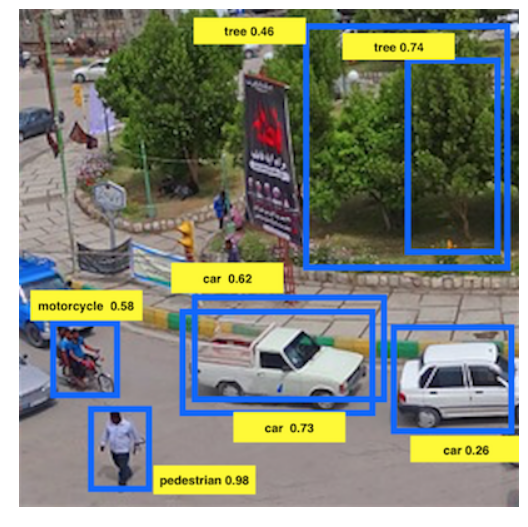Because the boundary of tree 0.74 and tree 0.46 did not overlap.

## Anchor boxes

Previously:
Each object in training image is assigned to grid cell that contains that object’s midpoint (and anchor box for the grid cell with highest IoU)
$$3 \times 3 \times 16 = 3 \times 3 \times 2 \times 8$$

## Region proposals (optional): R-CNN

Region - convolutional neural network
segmentation algorithm
N 2000 block, quite slow
R-CNN: propose regions. classify proposed regions one at a time. output label + bounding box
Fast R-CNN: Propose regions. use convolutional implementation of sliding windows to classify al the proposed regions
Faster R-CNN: use convolutional network to propose regions (slower that YOLO algorithm)

# Reference

 Redmon, J., Divvala, S., Girshick, R. and Farhadi, A., 2016. You only look once: Unified, real-time object detection. In Proceedings of the IEEE conference on computer vision and pattern recognition (pp. 779-788).

 Deeplearning.ai, Convolutional Neural Networks